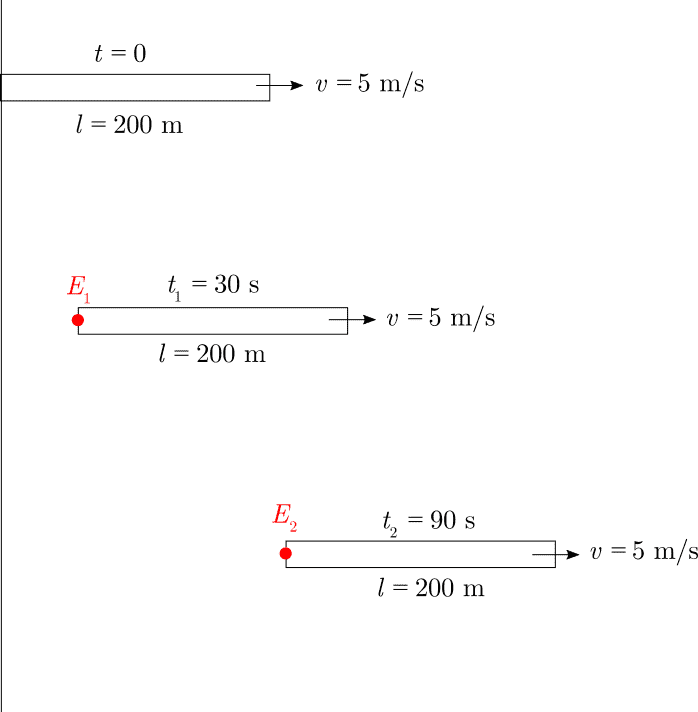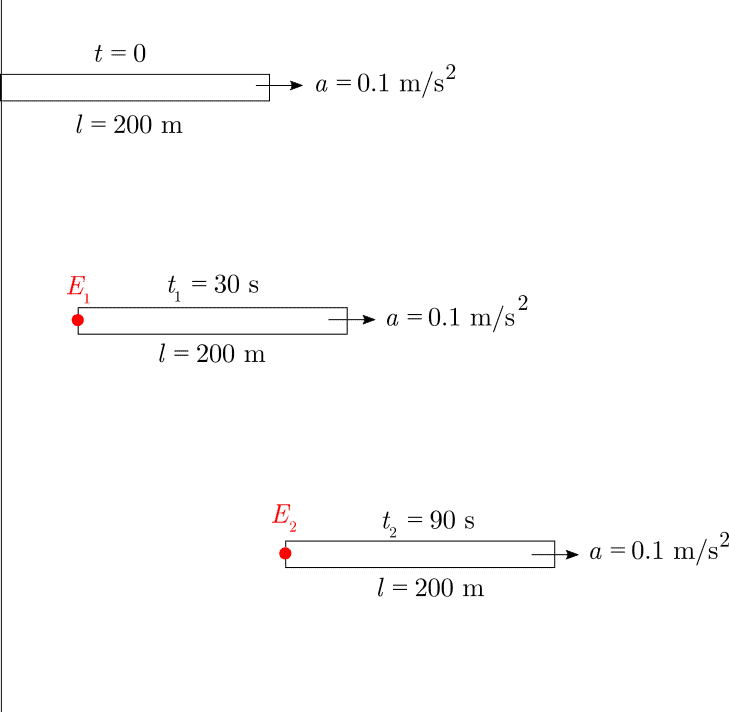# "Distance" between events in uniformly moving and accelerating frames

• brotherbobby
In summary, the conversation discusses the concept of uniformly moving frames and accelerating frames in relation to spacetime coordinates. Two methods are used to calculate the spatial separation between two events in the moving frame, with both methods yielding the same result. The concept of average relative velocity is also mentioned as a way to calculate the spatial separation between two events.

#### brotherbobby

Homework Statement
(1) A 200 m long goods train is moving uniformly with a speed of 5 m/s when its tail end crosses the front of the platform chosen to be the origin O. 30 s after is has passed, its tain signal flashes on and off, as seen by the platform observer. A further 60 s later, the same tail signal is observed to flash again. Calculate the distance between the two events as observed by the platform observer (##\Delta x##)and an observer on the train (##\Delta x'##).

(2) Carry out the solution to the same problem above if the goods train starts moving from the origin O from rest with a uniform acceleration of ##0.1 ms^{-2}##.
Relevant Equations
(1) According to Galilean transformations, for two frames in standard configuration along the ##x## axis, an event ##E## occuring at spacetime position ##(x, t)## in the frame S is observed to occur in frame S' at a spacetime position ##(x', t')## where (a) ##x' = x- vt## and (b) ##t'=t##, where ##v## is the (uniform) velocity of frame S' with respect to frame S along the positive ##x## axis.
(2) In the event of an acceleration of S' relative to S, but zero initial velocity (##v_0 = 0##)at ##t = t' = 0##, we have (a) ##x' = x+\tfrac{1}{2}at^2## and ##t'=t##.(1) Uniformly moving frames

I begin with a drawing of the situation. The events are labelled as ##\color{red}{E_1}## and ##\color{red}{E_2}##. We note the time of those events : ##t_1 = t'_1 = 30s## and ##t_2 = t_2' = 30+60 = 90s##.

I attempt the problem in two different ways.

(a) By calculating the spacetime coordinates of the events in the two frames

Since those events happen at the same location (the tail end) for the train observer, the spatial separation between them is 0. Hence the distance between the two events in the moving frame : ##\boxed{\color{blue} {\Delta x' = 0\,\text{m}}}##.
The location of event ##E_1## in the Earth's frame : ##x_1 = vt_1 = 5\times 30 = 150## m, from the origin. The location of event ##E_2## in the Earth's frame : ##x_2 = vt_2 = 5 \times 90 = 450## m. The spatial separation between the two events : ##\Delta x = 450 - 150 = 300## m. Hence the distance between the two events in the ground frame : ##\boxed{\color{blue}{\Delta x = 300\,\text{m}}}##.

(b) By direct use of the Galilean trasformations

According to the Galilean transformations, the location of the two events are related as ##x' = x-vt\Rightarrow x = x' + vt'##. (This is the same equation which relates the event's position in the S frame as a function of the the events coordinates in the S' frame brought about by replacing the velocity ##v## by ##-v## since S is moving with a velocity in the negative ##x-x'## direction relative to S').
For a pair of events, the above transformation leads to their spatial separation as ##\color{red}{\Delta x = \Delta x' + v\Delta t'}##, taking "differences" and remembering that velocity ##v## is constant. Thus, for the pair of events in our problem, assuming ##\boxed{\color{blue} {\Delta x' = 0\,\text{m}}}## and temporal separation ##\Delta t' = 60\,\text{s}##, we have ##\boxed{\color{blue}{\Delta x}} = 0 + 5\times 60 = \boxed{\color{blue}{300\,\text{m}}}##.

It looks good thus far, but this is where my confusions start, when the frame ##S'## accelerates.

(2) Accelerating frames(a) By calculating the spacetime coordinates

Since those events happen at the same location (the tail end) for the train observer, the spatial separation between them is 0. Hence the distance between the two events in the moving frame : ##\boxed{\color{blue} {\Delta x' = 0\,\text{m}}}##.
The location of event ##E_1## in the Earth's frame : ##x_1 = \tfrac{1}{2}at_1^2 = \tfrac{1}{2}\times 0.1\times 30^2 = 45## m. The location of event ##E_2## in the Earth's frame : ##x_2 = \tfrac{1}{2}at_2^2 = \tfrac{1}{2}\times 0.1\times 90^2 = 405## m. The distance between the two events in the Earth's frame : ##\boxed{\color{blue}{\Delta x}} = 405 - 45 = \boxed{\color{blue}{360\,\text{m}}}##.

(b) By using Galilean transformations for accelerated frames (my confusion scenario)

The Galiean transformations link the spacetime coordinates for the same event in two different frames S and S' in standard configuration along the ##x## axis, viz. ##x' = x-\tfrac{1}{2}a^2\Rightarrow x= x'+\tfrac{1}{2}at'^2##, where we "invert" the original equations and write the ##x## coordinate of the event in terms of the ##x'## coordinates, remembering that in the process ##a\rightarrow -a## as the S frame moves with an acceleration ##-a## with respect to S'. For the pair of events, "differencing" the above equation leads to ##\color{red}{\Delta x = \Delta x'+at'\Delta t'}##. Question is - ##\color{red}{\text{what is}\, t'?}##. There are two events, so which t' to take? If we take ##\color{red}{t'}## to be the ##\color{red}{\text{average}}## of the two times, we get ##t' = \tfrac{t'_1+t'_2}{2} = \tfrac{30+90}{2} = 60## s. Using this, we get the spatial separation as : ##\boxed{\color{blue}{\Delta x}} = \Delta x'+at'\Delta t' = 0 + 0.1\times 60\times 60 = \boxed{\color{blue}{360\,\text{m}}}##, which yields the same answer as in part (a) above.

Question is - does it make sense to take ##\color{red}{t'\, \text{as the average of the times between those of the two events}}##?

There is a different way to do this problem which takes into account the velocity of frame ##S'## at the time of event 1.

If we assume the spacetime location of event 1 to be the origin and try to find the location of event 2 relative to it, we observe that the frame ##S'## has a velocity relative to frame ##S## due to time of travel. Calling this velocity as ##v_0## and "starting" our clock with the timing of this event, the location of the second event is then given by : ##x_2 = x_2'+v_0 t_2' + \tfrac{1}{2} a{t'_{2}}^{2}##, where ##x'_2 = 0## since the 2nd event also happens to where we have shifted the origin and ##t' = 60##s, measured from the time of occurence of the first event. Hence, ##\boxed{\color{blue}{\Delta x}} = 0 + \underbrace{0.1\times 30}_{v_0} \times 60 + \tfrac{1}{2}\times 0.1\times 60^2 = 180 + 180 = \boxed{\color{blue}{360\,\text{m}}}##.

Are the two methods above correct, or is it that my reasoning errors lead to the correct answers for these pair of events?

•Delta2
brotherbobby said:
For the pair of events, "differencing" the above equation leads to ##\color{red}{\Delta x = \Delta x'+at'\Delta t'}##. Question is - ##\color{red}{\text{what is}\, t'?}##.

How did you get this equation?

It should be simply:
$$\Delta x = \Delta x' + \frac a 2 (t_2^2 - t_1^2) = \frac a 2 (t_2 - t_1)(t_2 + t_1) = \frac a 2 \Delta t (t_2 + t_1)$$

PeroK said:
How did you get this equation?

Imagine two variables linked by this equation : ##y=ax##. This implies that a change in ##y## is accompanied by a change in ##x## by ##\Delta y = a \Delta x##. LIkewise, if ##y = ax^2\Rightarrow \Delta y = 2ax\Delta x##.

Likewise, for a spacetime event viewed from different coordinate systems, we have the transformation ##x = x' +\tfrac{1}{2}at'^2\Rightarrow \Delta x = \Delta x' + 2\times \tfrac{1}{2} at' \Delta t' = \Delta x' + at' \Delta t'##.

(This is the same as in (1) above for the case of uniformly moving frames. We have ##x = x'+vt'\Rightarrow \Delta x = \Delta x' + v\Delta t'##)

Okay, you can use the concept of average relative velocity.

PeroK said:
Okay, you can use the concept if average relative velocity.

We have two events as above : ##E_1 (x_1, t_1)## and ##E_2 (x_2, t_2)##, their spacetime coordinates in the ##S## frame. Needless to say, the same two events have their own spacetime coordinates in the ##S'## frame, both primed to distinguish. These may or may not be the same as those in the ##S## frame.

In my equation above, the spatial separation or "distance" between the two events in the ##S## frame is given by ##\Delta x = \Delta x' + at'\Delta t'##. My confusion is about this quantity ##t'##.

I suppose what you meant in your quote above is that I can use the *average time* between the two events for ##t'## : ##t' = \frac{t'_1+t'_2}{2} \left(=\frac{t_1+t_2}{2}\right)##?

Even if I can, would it hold all the time? What is the acceleration ##a## was not uniform?

brotherbobby said:
Even if I can, would it hold all the time? What is the acceleration ##a## was not uniform?
No, the SUVAT formulas do not hold in that case.

PeroK said:
No, the SUVAT formulas do not hold in that case.
Yes thank you. Sorry for forgetting.